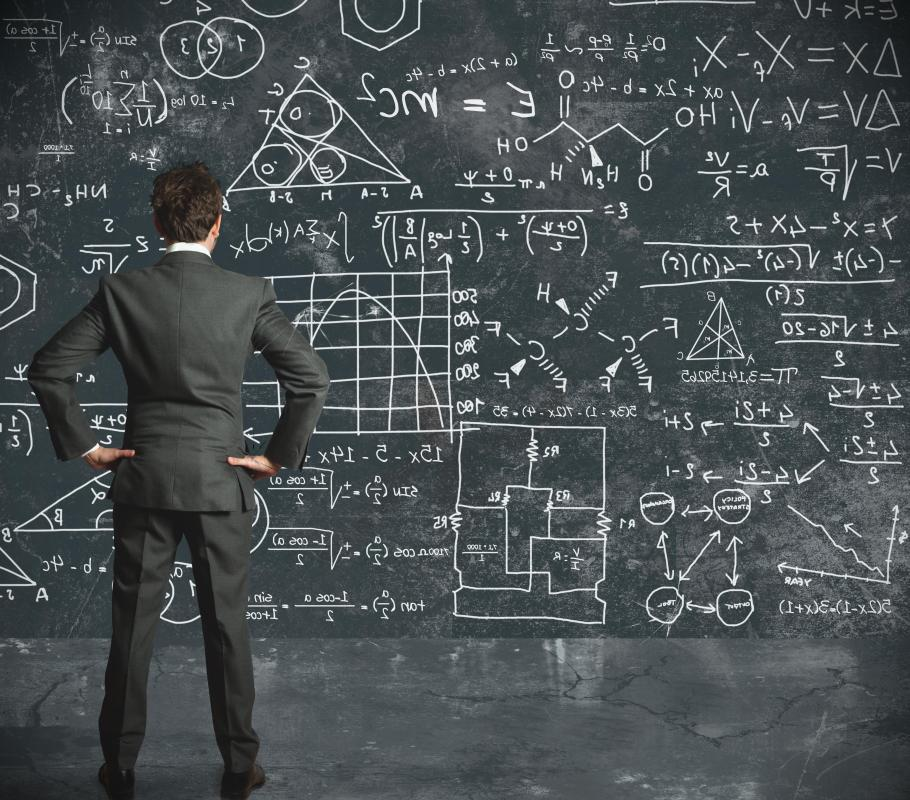# What Is Quantitative Physics?

Ray Hawk

Quantitative physics is the branch of physics that involves research by repeated measurement and mathematical analysis of experimental results. It differs from some branches of theoretical physics, for instance, such as quantum mechanics or string theory research, where much of the underlying theory cannot be tested in the real world, or in a laboratory on Earth with current technology as of 2011. Any field of quantitative research like quantitative physics derives its conclusions from a statistical analysis of large amounts of experimental data. This data is often so vast and complex, however, that computers are used to do mathematical modeling of the data to interpret it better. An example of the use of quantitative physics would include that of climate studies run on supercomputers to predict climatological changes from various natural thermodynamic forces at play on, in, or near the Earth, as well as from changes in solar activity over long periods of time.Quantitative physics is the branch of physics that involves research by repeated measurement and mathematical analysis of experimental results.

The study of physics at its core is the measurement of changes in matter and energy, and this makes most physics research quantitative physics in one form or another. Quantitative study is also important in physics because many of the physical laws, such as the speed of light or gravitational pull of the Earth, cannot be quantitatively defined just by human observation with the five senses. It is possible to observe a falling body, but, without precisely measuring its rate of descent, no clear picture is arrived at for how strong gravity actually is. Quantitative research physics, therefore, uses mathematics as an abstract way of understanding forces at work in the universe.Quantitative research physics uses mathematics as an abstract way of understanding forces at work in the universe.

Processes that involve quantitative study, however, are not always intended to represent everyday reality. Physics determines the ideal conditions under which matter, energy, space, and time interact through repeated measurement and observation, and then determines the probability of events occurring. Physics equations used for this are based on abstract mathematical concepts that are only proven true with large numbers of repeated experiments. Quantitative physics, for instance, can predict the surface area of a spherical planet in space, but there is no such thing as a perfect sphere or any other perfect geometric shape in the natural world, so the process is, to some degree, an approximation.

Ideal representations in physics, such as the ballistic trajectory of a bullet through the air, are based on quantitative physics principles of gravitational pull and air resistance, but they can only predict a general trajectory for a bullet, not the actual, precise spot upon which it will land. Using equations and formula in quantitative physics often involves averaging out some of the variables that come into play or using mathematical shortcuts to negate their effect on the equation. This is because the goal is to understand the laws of nature in principle over that of specific, random applications.

Computational physics often complements quantitative physics in the laboratory, where equations cannot be formally or adequately tested in real world experiments. Often algorithms are used to streamline such calculations. Algorithms are a set of mathematical rules that the computer uses to reduce the number of calculations needed to solve a problem down to a finite series of steps. Computer assistance for quantitative physics is usually employed in areas where very complex interactions take place, such as in materials science, nuclear accelerator research, and molecular dynamics in biology.

## You might also Like